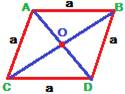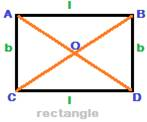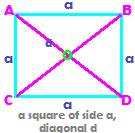## Perimeter and area

The perimeter and area formulas for various plane figures are pithily tabulated below.
The plane figures are:

Trapezium, Parallelogram, Rhombus, Rectangle, Square.

The formulas for perimeter and area are given in the table below for quick reference without solving any problems on them.

 Quadrilateral/ Plane Figure Perimeter Area Trapezium AB + BC + CD + AD Parallelogram AB + BC + CD + AD            =       2(b +h) base × height b × h RhombusAB + BC + CD + AD = 4a a is side ½ ×(product of diagonals) Rectangle2 × ( l + b) l × b Square4a a is side a2 or d2/2 d is diagonal length